﻿ 舰船辐射信号中压缩感知降噪性能的研究
 舰船科学技术2017, Vol. 39Issue (10): 112-116PDF

Research on noise reduction function of compressed sensing in ship-radiated noise
NING Wan-zheng
Jiangsu Automation Research Institute, Lianyungang 222006, China
Abstract: For the problem of signal with noise in Compressed Sensing (CS), it is studied that, in the compression sampling process, the information of the pure signal is retained, but the information of the noisy signal is compressed and lost. Thereby the CS itself has the function of noise reduction. Taking the ship radiation signal with Gaussian noise as an example, when the Signal to Noise Ratio (SNR) is less than 20 dB, there is always a reasonable number of measurements to make the Mean Square Error (MSE) between reconstruction signal and pure signal is lower than the MSE between noisy signal and pure signal. So, it can increase the SNR indirectly.
Key words: ship-radiated noise     compressed sensing     noise reduction
0 引 言

1 压缩感知的基本理论

1）原始信号X在基底 $\varPsi\left( {N \times N} \right)$ 下稀疏，稀疏系数向量为Θ

 $X = \mathop \sum \limits_{i = 1}^N {\theta _i}{\psi _i}\;{\rm{or}}\;X = \varPsi \varTheta \text{，}$ (1)

2）利用观测矩阵 ${ \varPhi} \left( {M \times N} \right)$ 获取观测值Y

 $Y = { \varPhi} X = \varPhi \varPsi \varTheta = {A^{CS}}\varTheta \text{，}$ (2)

3）已知 ${ \varPhi} ,\varPsi ,Y$ ，选择合适的算法恢复X

 $\hat X = {{ \varPhi} ^{ - 1}}Y\text{，}\left\{ {\text{满足}Y = {A^{CS}}\varTheta \text{的最稀疏解}} \right\}\text{。}$ (3)

Candès和Tao在文献中指出，信号如果在某领域的变换系数满足具有幂次速度衰减，就可以用压缩感知理论得到恢复，同时指出，光滑信号的Fourier系数、小波系数、离散余弦系数等都具有足够的稀疏性，可以通过压缩感知理论恢复信号。如果变换系数的非零个数（或绝对值较大的系数个数）为K，则称信号为K-稀疏信号，K为信号的稀疏度。

 ${\rm min}{\varPsi ^{\rm T}}{X_p}\;\;s.t.\;\;\;Y = {\Phi} \varPsi \varTheta \text{，}$ (4)

2 舰船辐射噪声的稀疏性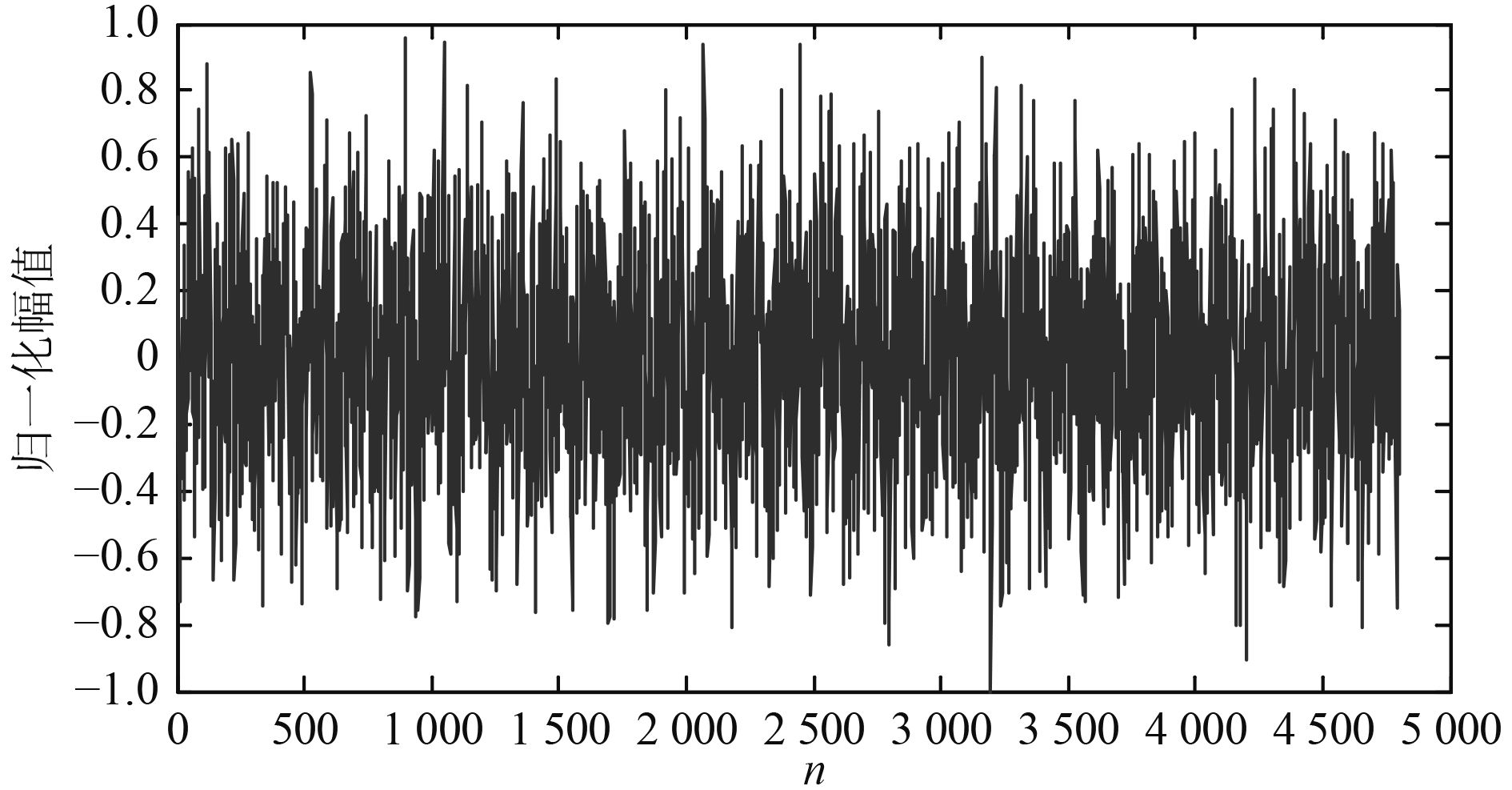图 1 某舰船辐射噪声信号时域波形 Fig. 1 Time-domain waveform of ship-radiated noise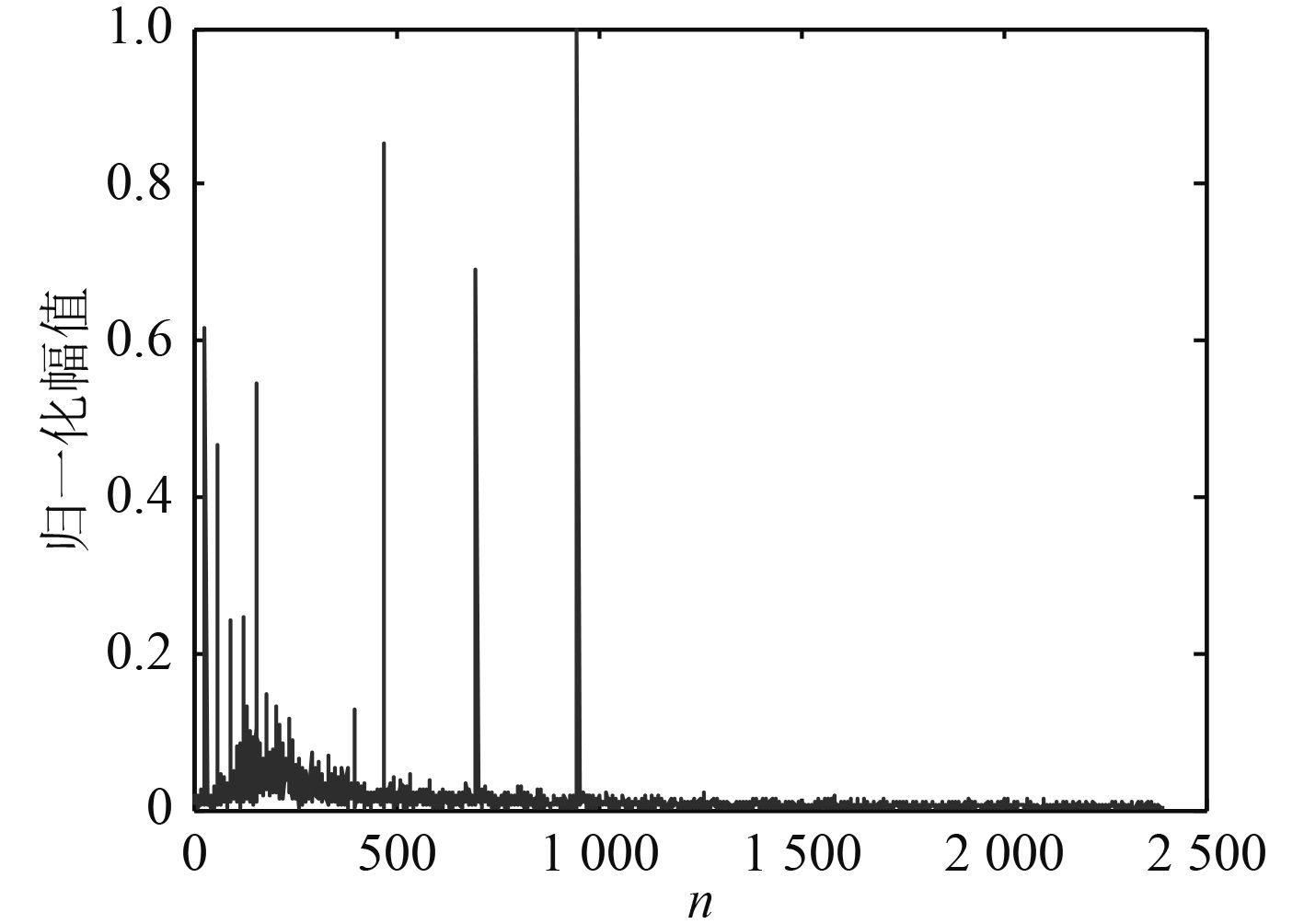图 2 舰船辐射噪声的频域波形 Fig. 2 Frequency-domain waveform of ship-radiated noise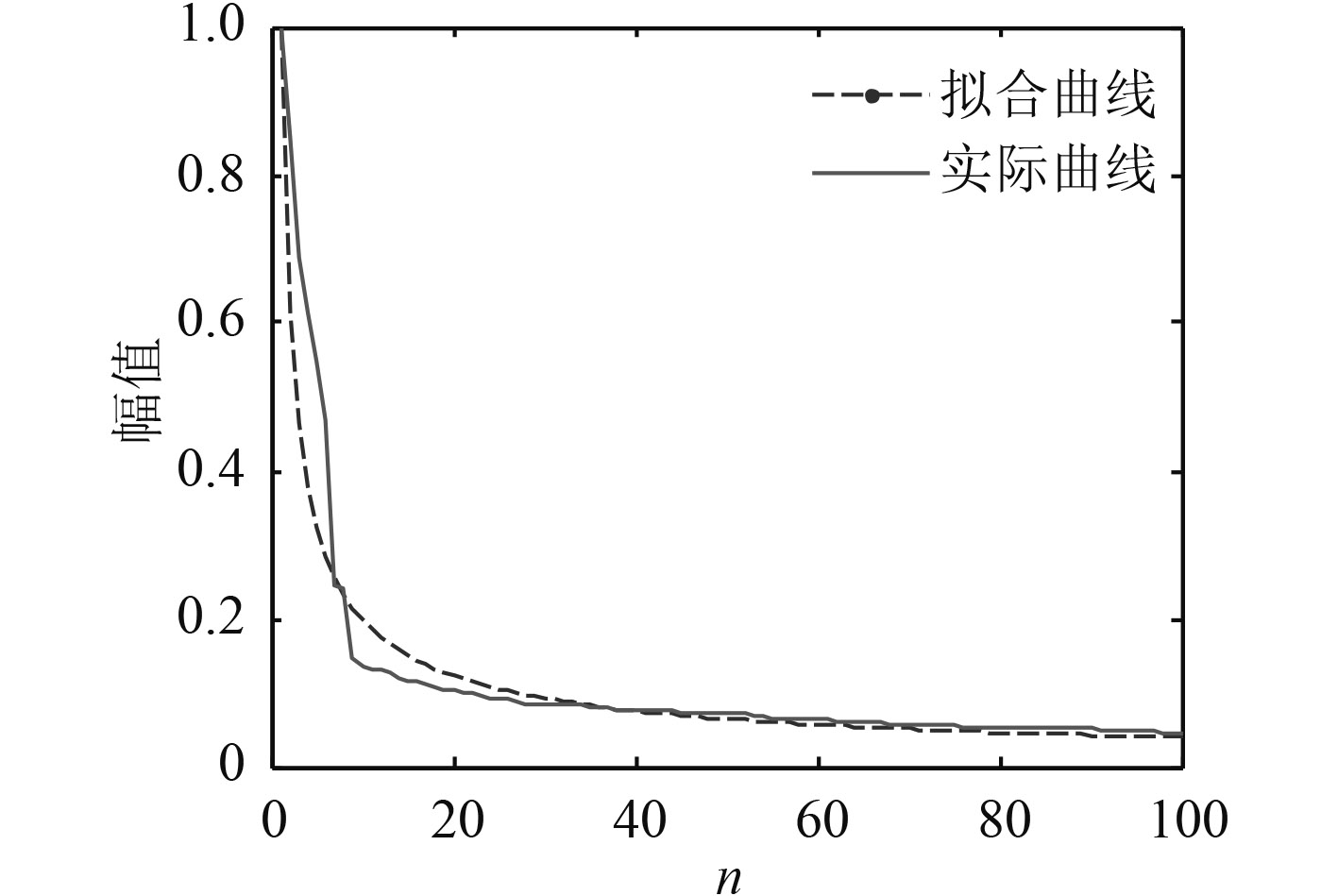图 3 舰船辐射噪声FFT系数衰减曲线 Fig. 3 FFT attenuation of ship-radiated noise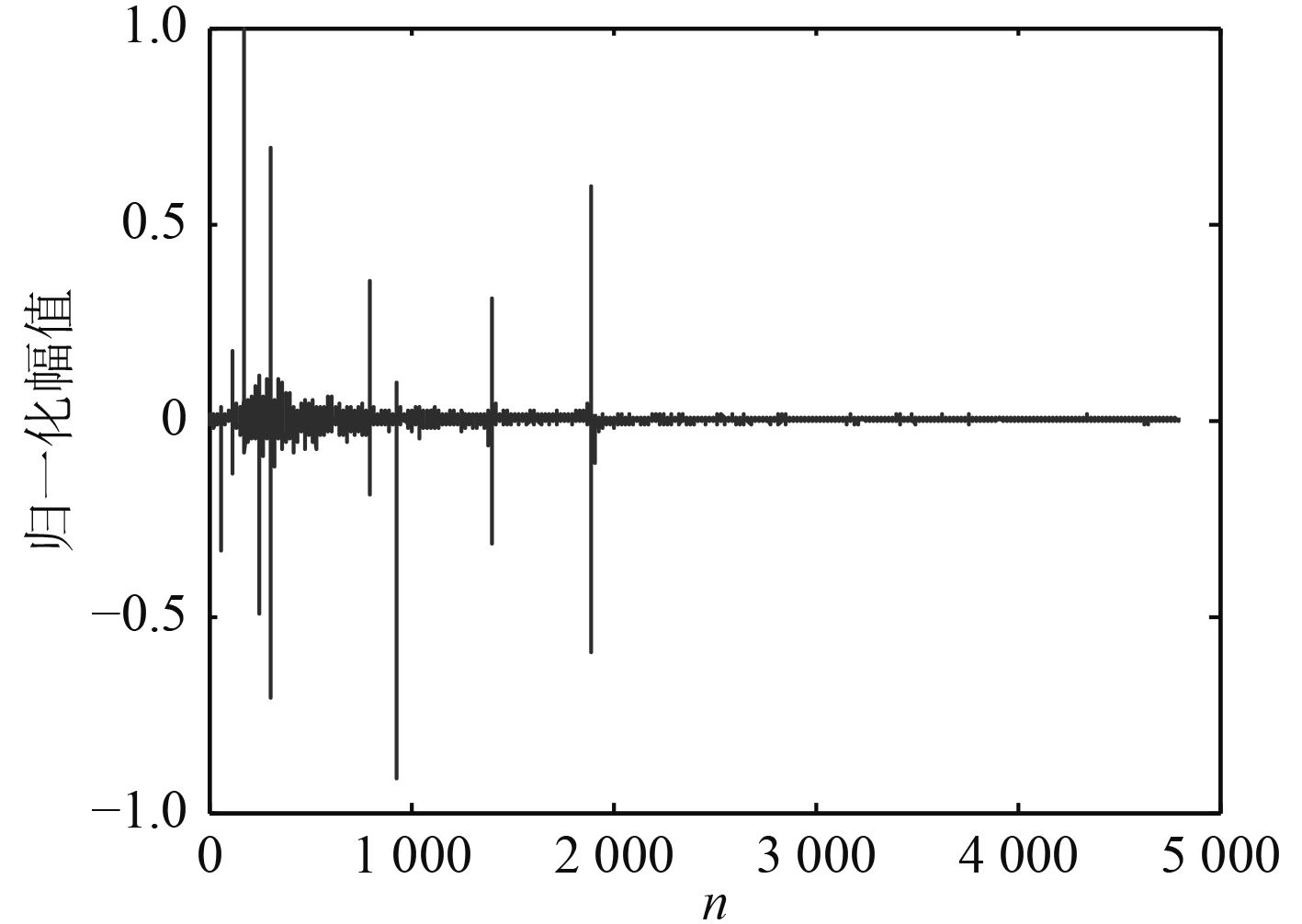图 4 舰船辐射噪声的DCT波形 Fig. 4 DCT waveform of ship=radiated noise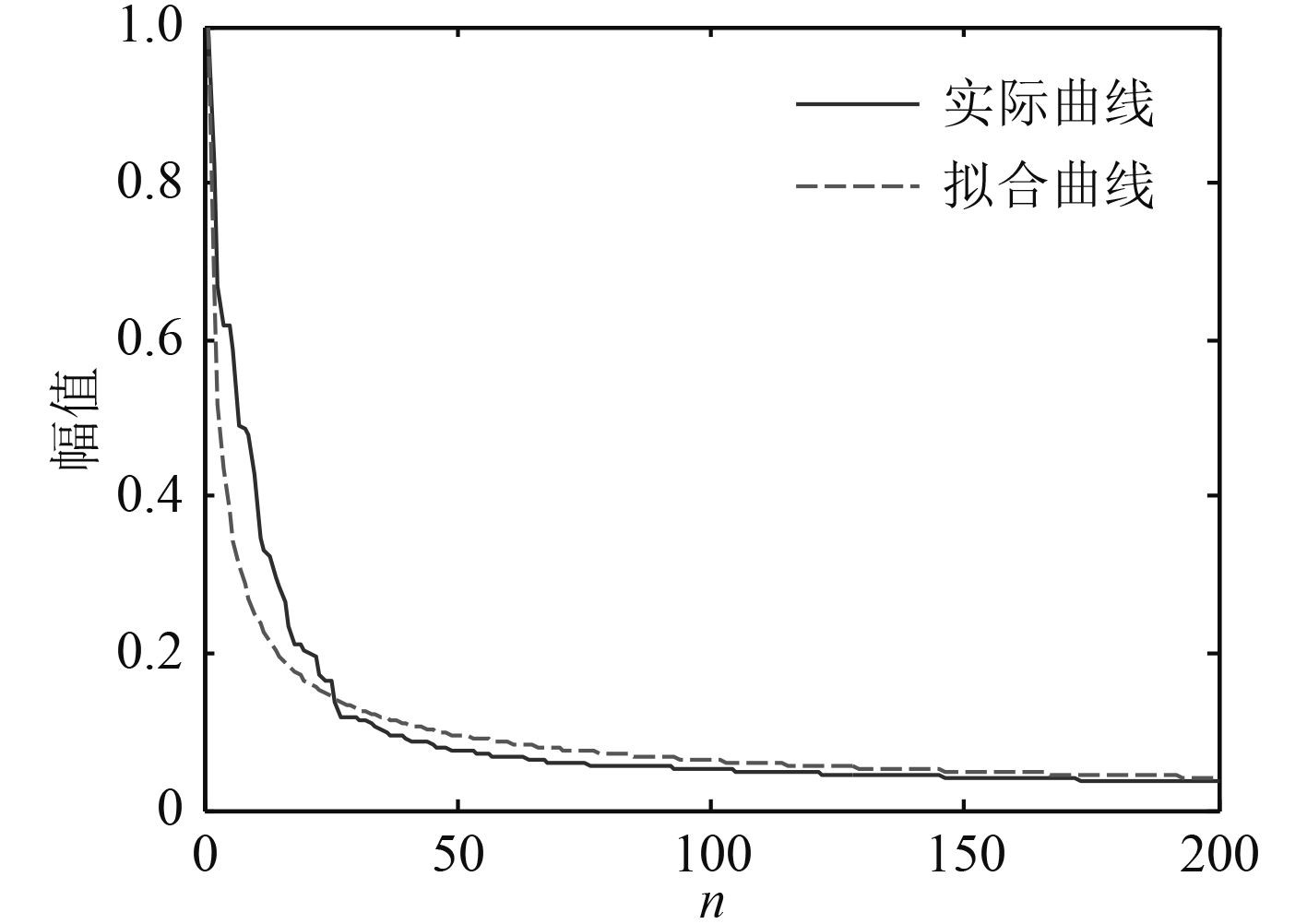图 5 舰船辐射噪声DCT系数衰减曲线 Fig. 5 DCT attenuation of ship-radiated noise

3 压缩感知的降噪性能研究

 $S = X + {N_0}\text{，}$ (5)

 $\begin{array}{l}S = X + {N_0} = \mathop \sum \limits_{{\rm{i}} = 0}^N \left( {{\theta _i}{\varphi _i} + {\theta _{0i}}{\varphi _i}} \right)\text{，}\\\;\;\text{或者}S = X + {N_0} = \varPsi \left( {\Theta + {\Theta _0}} \right)\text{。}\end{array}$ (6)

 $\hat Y = { \varPhi} X + {N_0} = {A^{CS}}\varTheta + {A^{CS}}{\varTheta _0}\text{，}$ (7)

4 仿真试验

1）一定信噪比、一定观测值数目下的信号重构情况仿真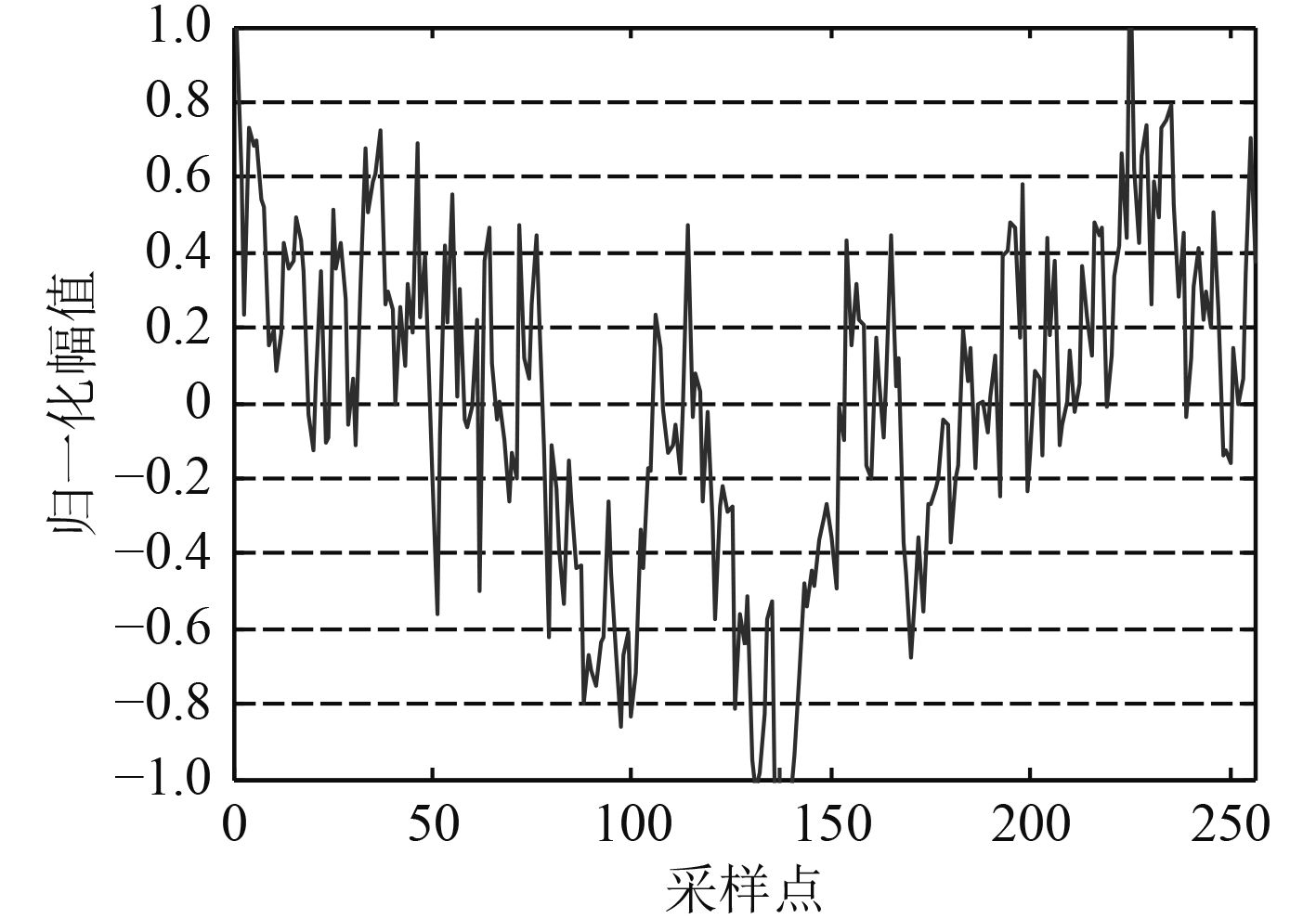图 6 SNR=5 dB的舰船辐射信号 Fig. 6 The ship-radiated noise when SNR=5 dB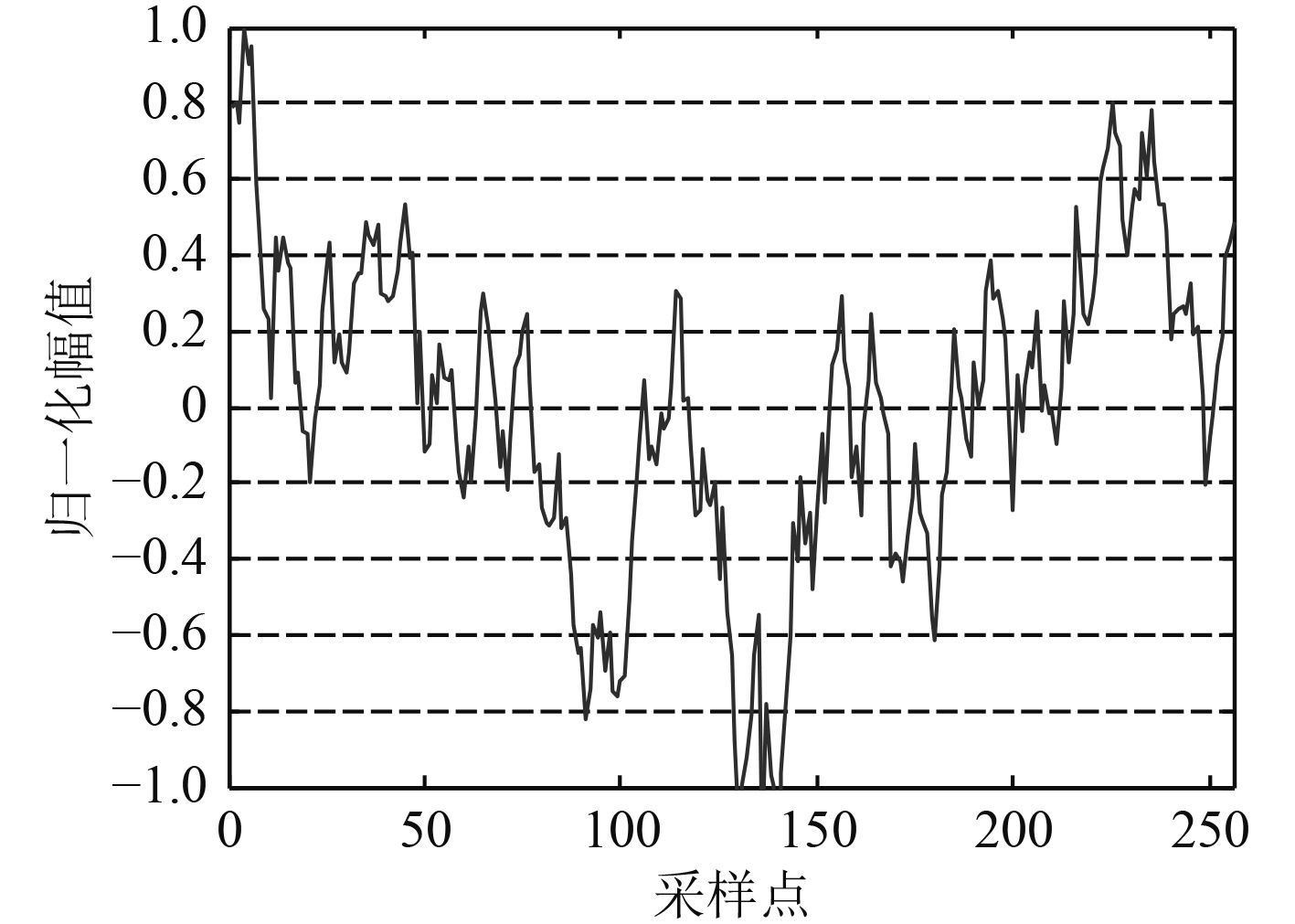图 7 SNR=10 dB的舰船辐射信号 Fig. 7 The ship-radiated noise when SNR=10 dB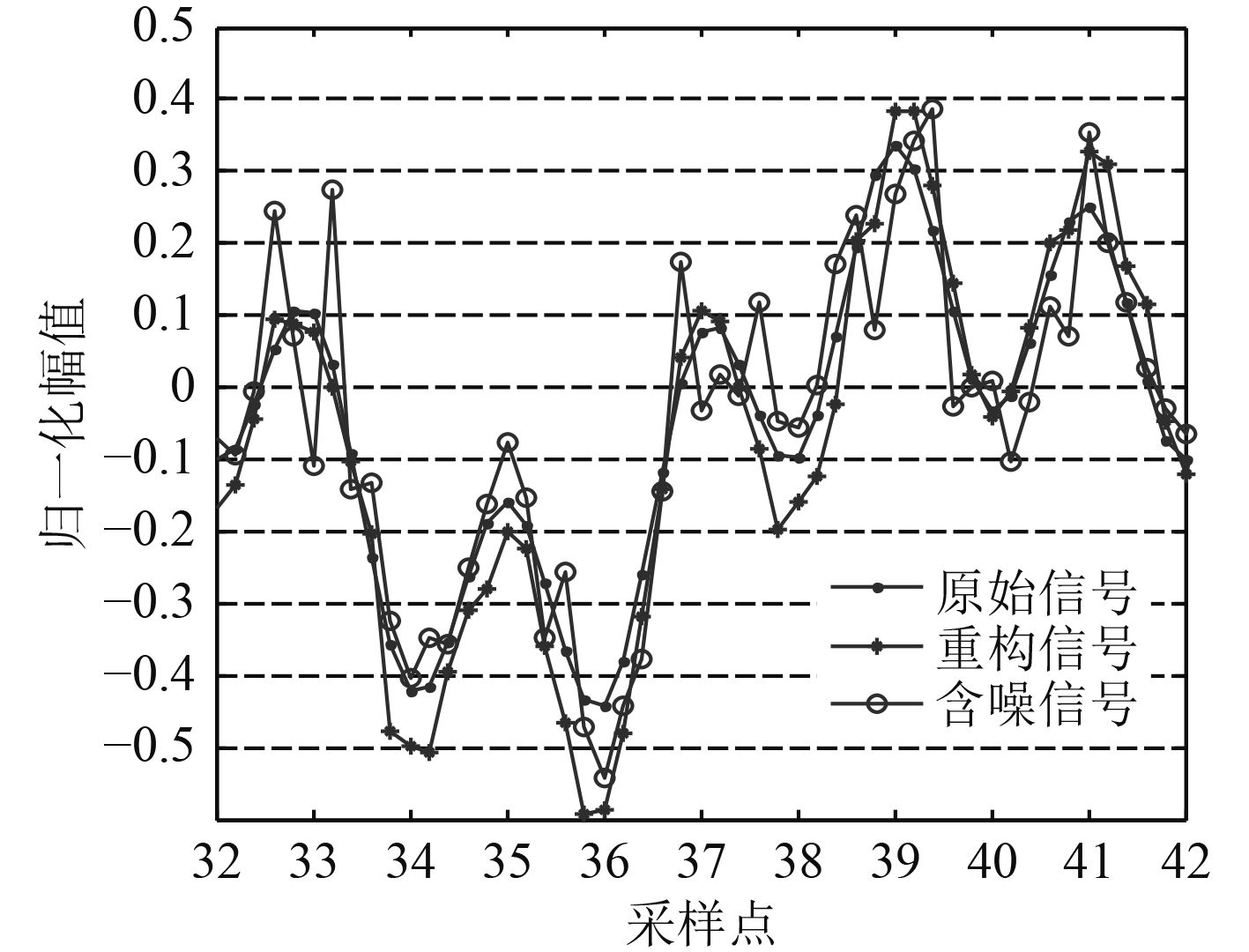图 8 SNR=5 dB M=58时的重构性能 Fig. 8 The reconstruction effect when SNR=5 dB and M=58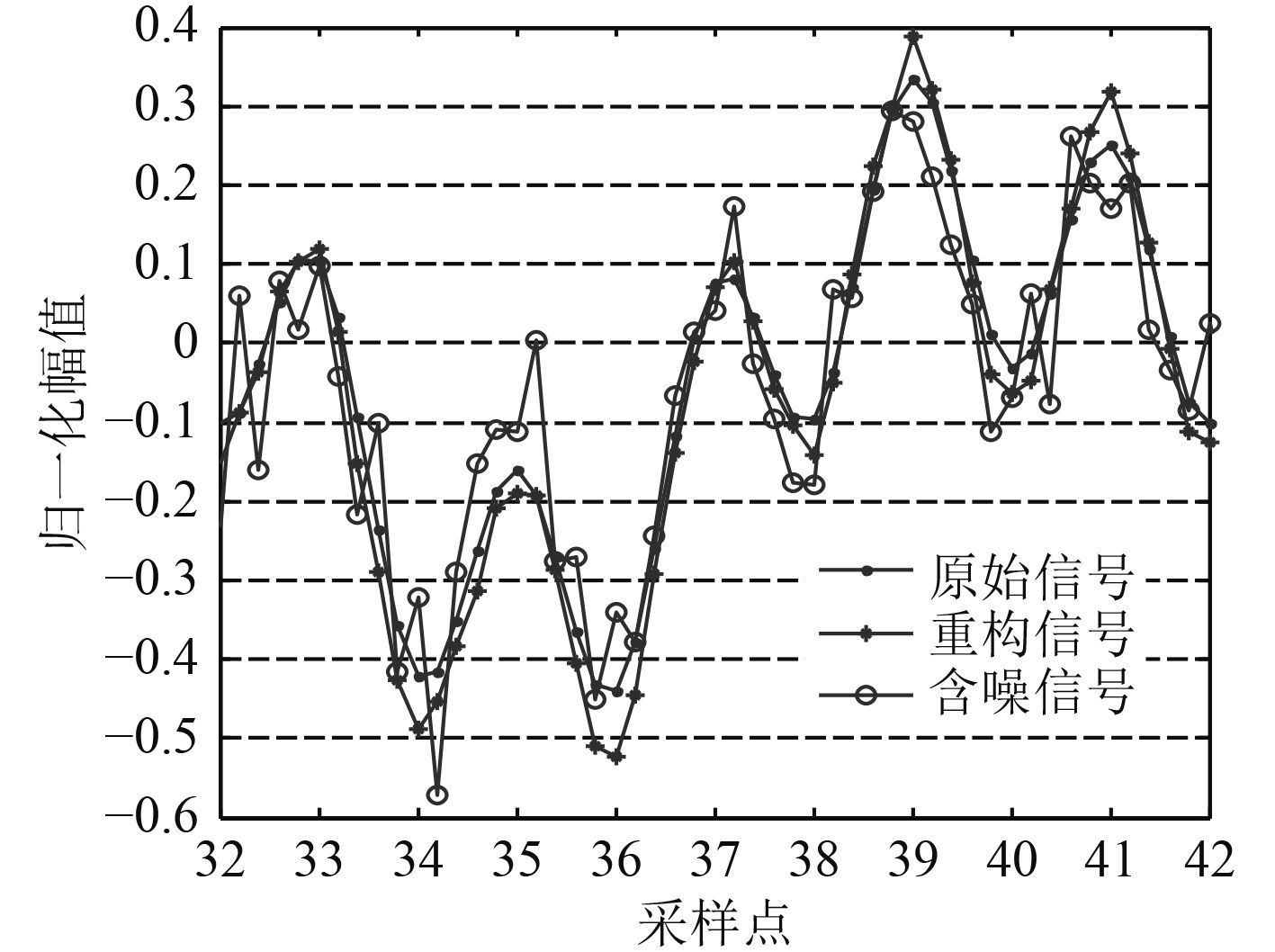图 9 SNR=10 dB M=62时的重构性能 Fig. 9 The reconstruction effect when SNR=10 dB and M=62

2种情况下，观测值数目均小于M0，重构信号的均方误差也都小于含有背景噪声的信号误差，即重构信号比含噪信号本身更接近原始信号，压缩感知过程间接起到了提高信噪比的作用。这显然有利于舰船辐射噪声的检测与跟踪。

2）不同信噪比下，重构效果随观测值数目变化趋势的仿真

X为原始信号， ${{\hat X}}$ 为重构信号，对两者各元素取绝对值后，分别记为X’， ${\rm{\hat X'}}$ ，则匹配度定义式如下：

 ${Mat}_{rate} = 1 - \frac{{{{{{\hat X}}}^{\rm{'}}} - {{{X}}^{\rm{'}}}_2}}{{{{{{\hat X}}}^{\rm{'}}} + {{{X}}^{\rm{'}}}_2}}\text{，}$ (8)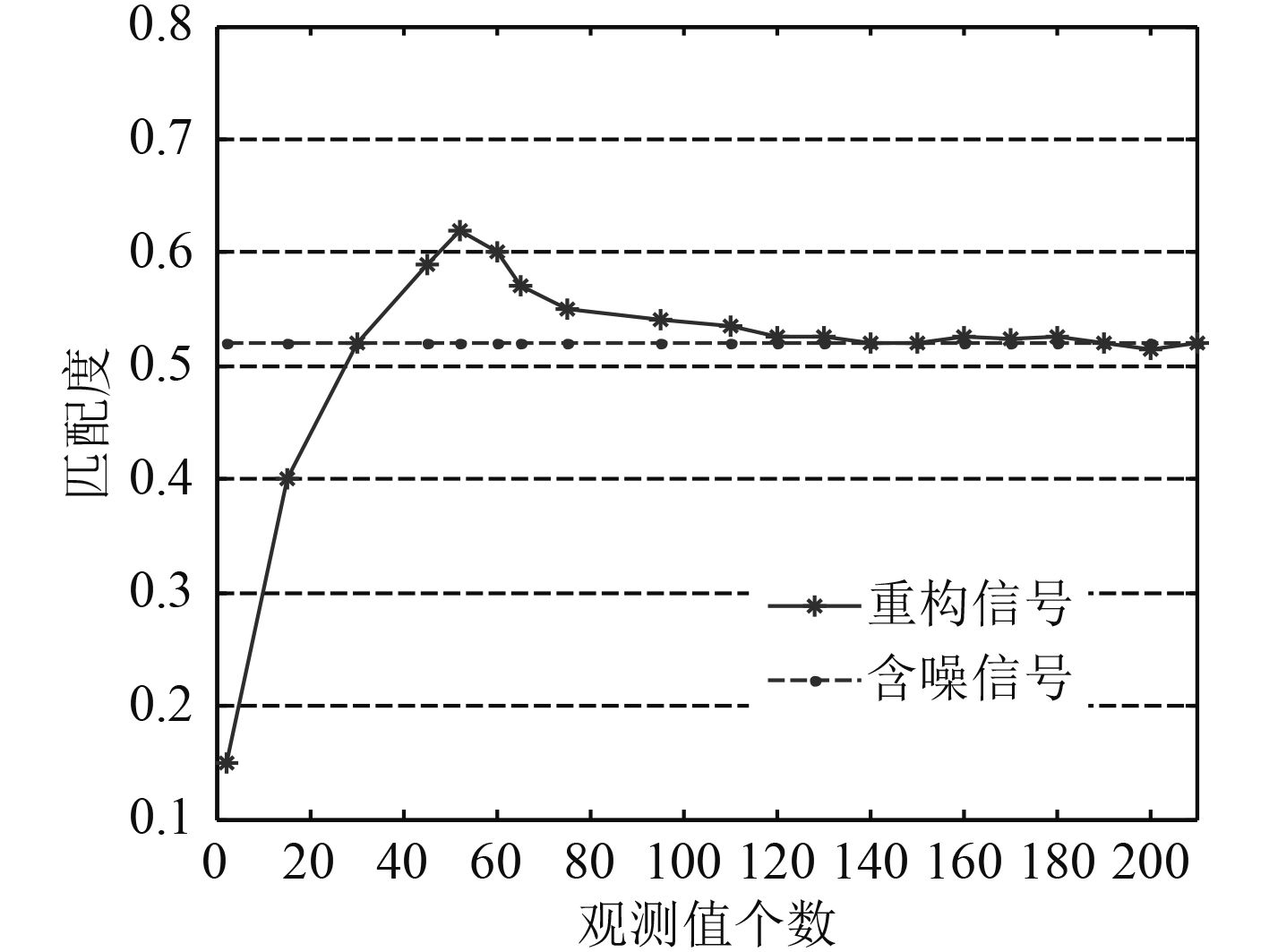图 10 SNR=0 dB时匹配度随观测值变化曲线 Fig. 10 MR cure along with the number of measurements when SNR=0 dB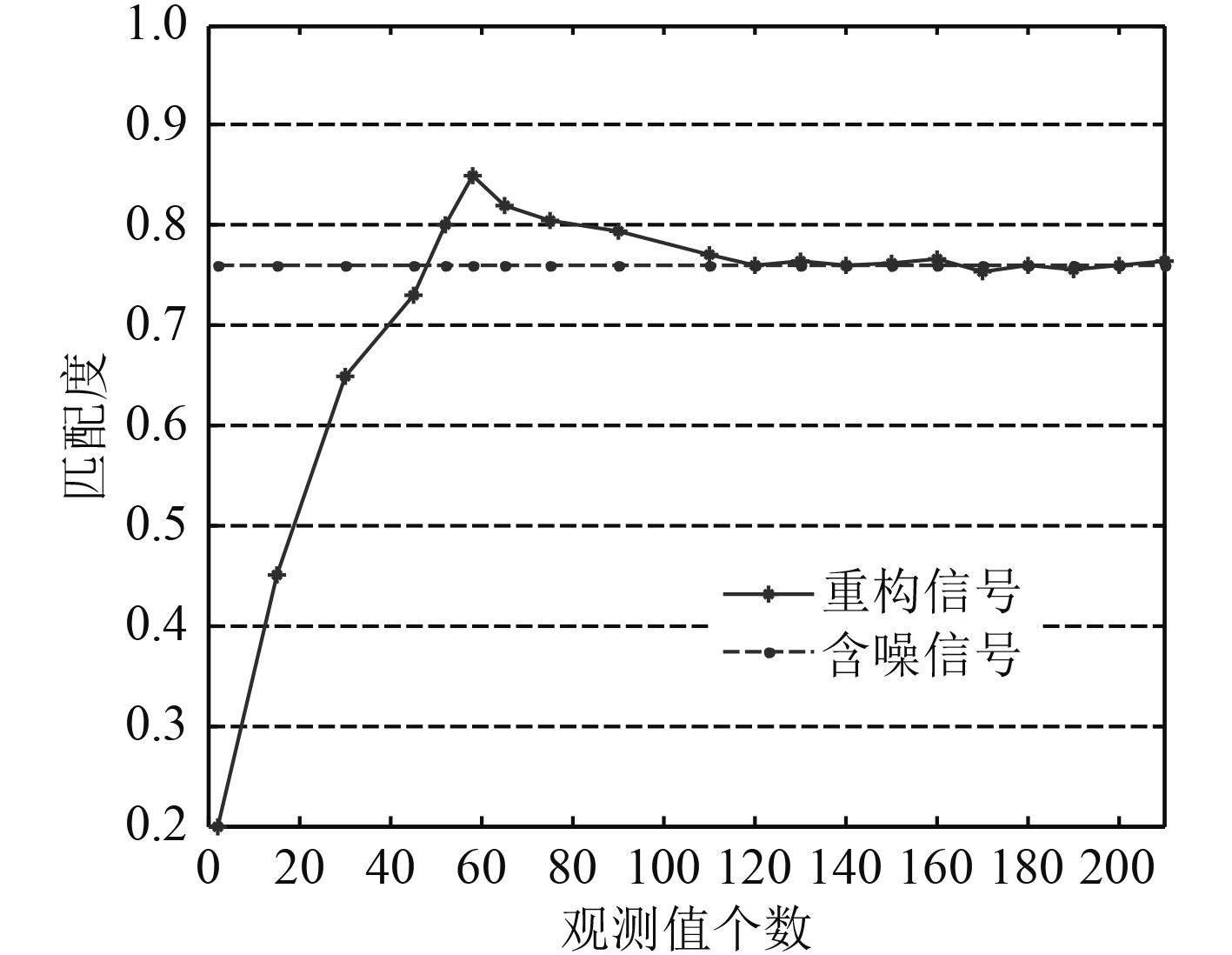图 11 SNR=5 dB时匹配度随观测值变换曲线 Fig. 11 MR cure along with the number of measurements when SNR=5 dB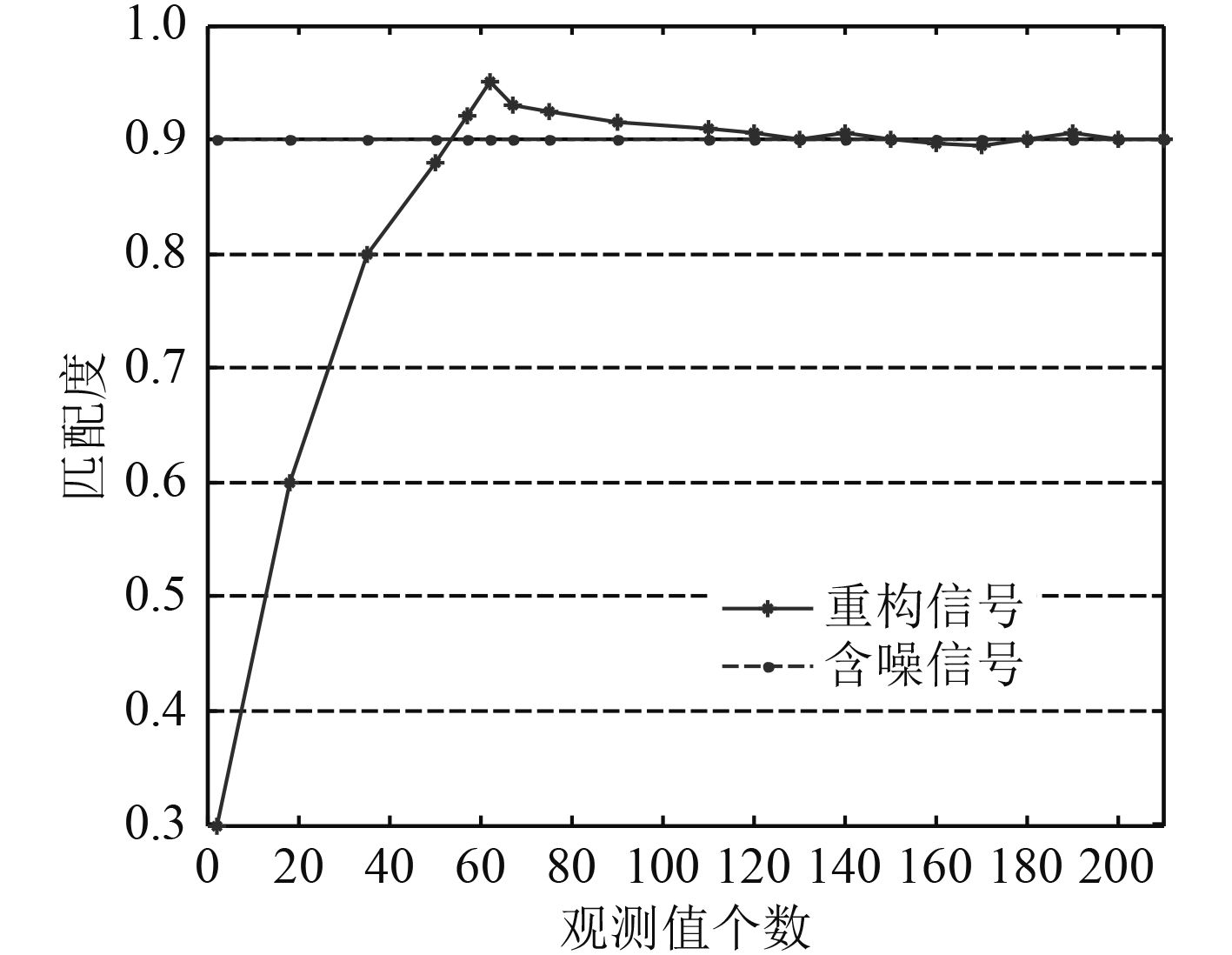图 12 SNR=10 dB时匹配度随观测值变化曲线 Fig. 12 MR cure along with the number of measurements when SNR=10 dB图 13 SNR=20 dB时匹配度随观测值变换曲线 Fig. 13 MR cure along with the number of measurements when SNR=20 dB

5 结 语

  BARANIUK R. A lecture on compressive sensing[J]. IEEE Signal Processing Magazine, 2007, 24 (4): 118–121. DOI: 10.1109/MSP.2007.4286571  E CANDÈS, J ROMBERG. Robust uncertainty principles: Exact signal reconstruction from highly incomplete frequency information[J]. IEEE Trans on Information Theory, 2006, 52 (2): 489–509. DOI: 10.1109/TIT.2005.862083  D L DONOHO. Compressed sensing[J]. IEEE Trans on Information Theory, 2006, 52 (4): 1289–1306. DOI: 10.1109/TIT.2006.871582  D NEEDELL, J A TROPP. CoSaMP: Iterative signal recovery from incomplete and inaccurate samples[J]. Appl Comp Harmonic Anal, 2009, 26 (3): 301–321. DOI: 10.1016/j.acha.2008.07.002  LI Xiao-bo. The research of measurement matrix based on compressed sensing[D], Beijing: Beijing Jiaotong University, 2010.  周灿梅. 基于压缩感知的信号重建算法研究[D]. 北京: 北京交通大学, 2010.